## 1. Overview

Many ways exist to compute the area of geometric shapes using mathematical formulas. Here, we will focus only on 2D polygons. A polygon consists of a finite number of connected segments that we can represent as a set of consequential points.

There are different formulas to compute the area of regular polygons and several techniques for decomposition. However, the area of non-regular polygons is a bit hard to compute.

In this tutorial, we’ll learn how to calculate the area of an arbitrary 2D polygon using some basic operations from linear algebra.

## 2. Main Definitions and Operations

First, we must understand a few concepts about 2D vectors and matrices.

### 2.1. Vector

A vector in a 2D space is a geometric object described by its magnitude and direction or. For example, we can view a vectoras the displacement from pointto point: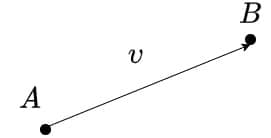The figure above represents a vector described by two pointsandwith coordinatesandrespectively. Then, the vector is.

### 2.2. Matrix and Determinant

A matrix is a 2D array of numbers withrows andcolumns. When, the matrix is square and has a determinant, a unique value that characterizes the matrix.

To compute the determinant of amatrix, we should multiply the top-left and bottom-right elements of the matrix (also called the main diagonal). Then, subtract from this the product of the bottom-left and top-right elements:## 3. Area of Triangle

Let’s start by computing the areas of a triangle, and a parallelogram, then extend this to other geometric shapes. Linear algebra provides straightforward formulas to calculate the area of triangles and parallelograms if we know the coordinates of all the vertices on the 2D plane.

So, suppose we have a parallelogram: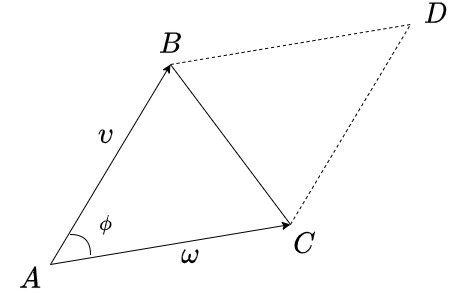The area of a parallelogram is. Alternatively, the area is also equivalent to the determinant of a square matrix with vectorsandas columns:.

Consequently, the area of the triangle is:.

For example, let’s compute the area of the following triangle: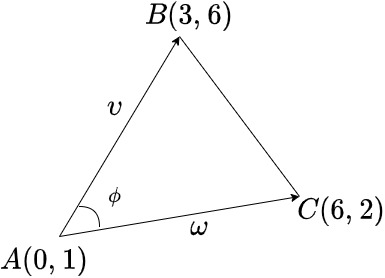..

Here, clockwise ordered vectorsandyield a negative area. Instead, counter-clockwise vectors return a positive area:.

This formula for area is a very efficient computation as it doesn’t involve roots or trigonometric functions. Instead, there are just two multiplications, five additions, and possibly one division by two.

## 4. Area of Polygon

Let’s use this understanding on a generic 2D Polygon.

### 4.1. Idea of Computation

First, a simple polygon withvertices has no self-intersections and can be decomposed intotriangles. We can calculate the signed areas of each triangle and then add them together to calculate the total area of the polygon. As seen previously, the resulting area can be either positive or negative depending on the order of evaluation.

Mathematically speaking, givenan arbitrary point on the 2D space andthe vertices of the polygon andto close the polygon. We can compute the area as the sum of all areas of triangles.

### 4.2. Why It Works

Let’s look at the example of the polygon and point: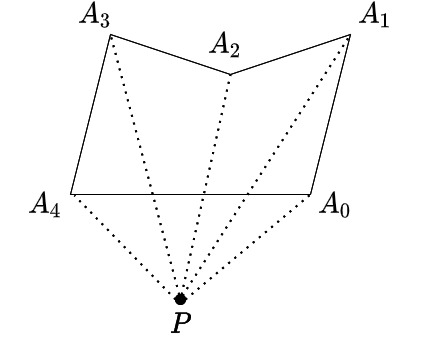We have to calculate the area of five triangles:.

Above, we evaluate the orientation of the first four triangles counter-clockwise and the last one clockwise. Therefore, the area of the first four triangles must be positive, and the last one must be negative. This difference will remove the extra area not contained in our polygon. The extra space, in our case, is precisely the triangle:.

### 4.3. Formula to Compute the Polygon Area

We may choose a specific pointto simplify the final computation formula. Assume we have a polygon represented with the set ofpoints. Thus, the formula that computes the area of the polygon:, wherewith.

Above is the sum of all triangle areas formed with each line segment of a polygon. Remember, we have to include all polygon edges, and the last triangle of the sum will be triangle. Thus, the index.

## 5. Green’s Formula

Finally, let’s prove the above formula. Referring to mathematical analysis, we should know about Green’s Theorem.

Letbe our polygon line segments. Letbe a region bounded with. Noticeis our target region. The goal is to compute its area. Supposeandare functions, defined on. Then, Green’s Theorem states:So, in order to compute the area of polygon, we need to chooseandsuch that:. The appropriate choice isand. Thus, ifis our target polygon area, the formula transforms to.

Importantly,is our polygon line segmentsand. And the area:. Also, we assume all line segments are oriented counter-clockwise.

To compute each integral in the sum, we can represent each line segment:,,.

The last thing that we need to do is to substitute the parameterization:.

And the final formula that computes the target polygon area:.

## 6. Example of the Polygon Area Calculation

Let’s use the formula just described and calculate the area of the polygon the following polygon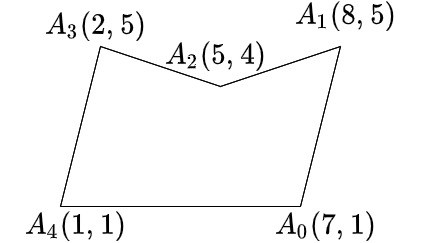If we choose the point, then the area is:To emphasize, notice that our target pointis on the bottom-left of our polygon. As a consequence the areas ofare positive and the areas ofare negative. As expected, the extra computed area is exactlyand.

## 7. Conclusion

In this article, we’ve introduced a way to calculate the area of a 2D polygon. Furthermore, we’ve touched on some major topics of linear algebra. Moreover, we also introduced methods to calculate the area of the triangle and parallelogram, as they are specific polygons.